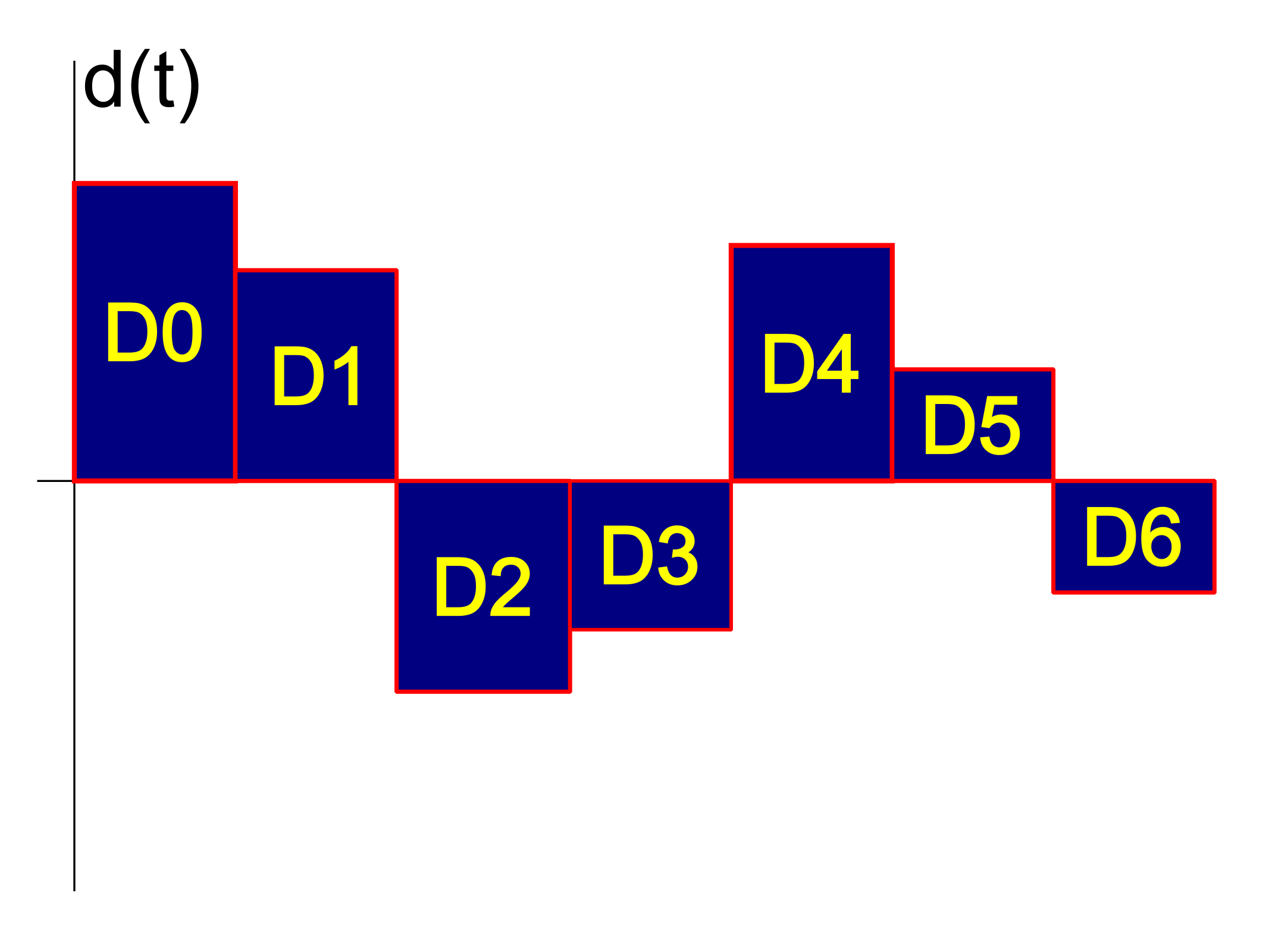# Dual DAC CTSD | Wider Bandwidth and Higher SNR — Part 2

## Introduction

1. A low-precision tight loop that delivers the first sample to the quantizer
2. A higher-precision loop that goes through a clock delay to minimize “metastability” (indecision)

## DAC Feedback

As a small aside, recall that the DAC feedback looks similar to the following:The input to the quantizer is the convolution of this signal with the impulse response h(t) of the noise-shaping filter H(s). As we did with the impulse-invariant transform, we break down the noise-shaping filter into partial-fraction expansion:

H(s) = \displaystyle \sum_{k} F_{k}(s) = \sum_{k} \frac{g_k}{s - p_k}

Each of these F_{k} is a single-pole filter, with an impulse response that looks something like:## Dual-Filter Analysis

The first sample at the quantizer is the convolution of a DAC symbol with the impulse response. This amounts to the integral over one sample period:The rest of the samples can be passed through a filter that looks like this:The main difference between this remaining filter and the original is that it is scaled by A_{1} = e^{-p_{k}T_s}. Note that the delay in the filter is already included in the system due to the extra clock delay by the second DAC.

## Summary

So, in the end, we have replaced H(s) with:

H'(s) = \displaystyle \sum_k e^{-p_{k}T_s}F_{k}(s)